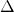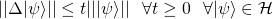## David Davalos, PhD

Mathematical Physicist, Applied Mathematician (Data Science, Machine Learning) and Developer (Python)

# Playing with math sym.

Something interesting must come here soon. This webpage is possible thanks to the support by Armando.

Letan operator over the Hilbert space, we call it bounded if and only if, with. The set of all bounded operators overis denoted as. In particular the minimumfor which the inequality above holds, is called the operator norm of. This norm induces the operator norm topology. Such norm is typically too strong for many purposes, a very wide used one is the so called weak operator topology, it will be discussed later.